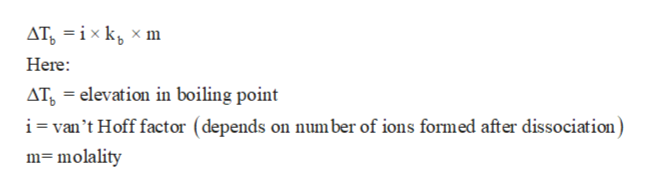# Calculate the boiling point of 178g of CaCO3 dissolved in 1kg of water?

Question
2 views

Calculate the boiling point of 178g of CaCO3 dissolved in 1kg of water?

check_circle

Step 1

Colligative properties are the properties which depend on the number of atoms or molecules or ions or particles present in the solution. Some common colligative properties are depression in freezing point, elevation in boiling point, osmotic pressure and relative lowering in vapor pressure.

Step 2

Elevation in boiling point is a colligative property that can be calculated with the help of concentration (molality) of solution. Hence as the number or moles of solute increases, the boiling point also increases. The relation between elevation in boiling point and molality of solution can be written as:help_outlineImage TranscriptioncloseAT ixk x m Here ATelevation in boiling point i=van't Hoff factor (depends on number of ions formed after dissociation) mmolality fullscreen
Step 3

Given:

Mass of CaCO3 = 178g

Mass of water = 1 kg

Molar mass of CaCO3 = 100.1 g/mol

Kb for water = 0.52 K .kg mol-1

The value of i for CaCO3 = 2 (as CaCO3 gives ...

### Want to see the full answer?

See Solution

#### Want to see this answer and more?

Solutions are written by subject experts who are available 24/7. Questions are typically answered within 1 hour.*

See Solution
*Response times may vary by subject and question.
Tagged in

### Solutions